# 算法原理¶

## 1. Quantization Aware Training量化介绍¶

### 1.1 背景¶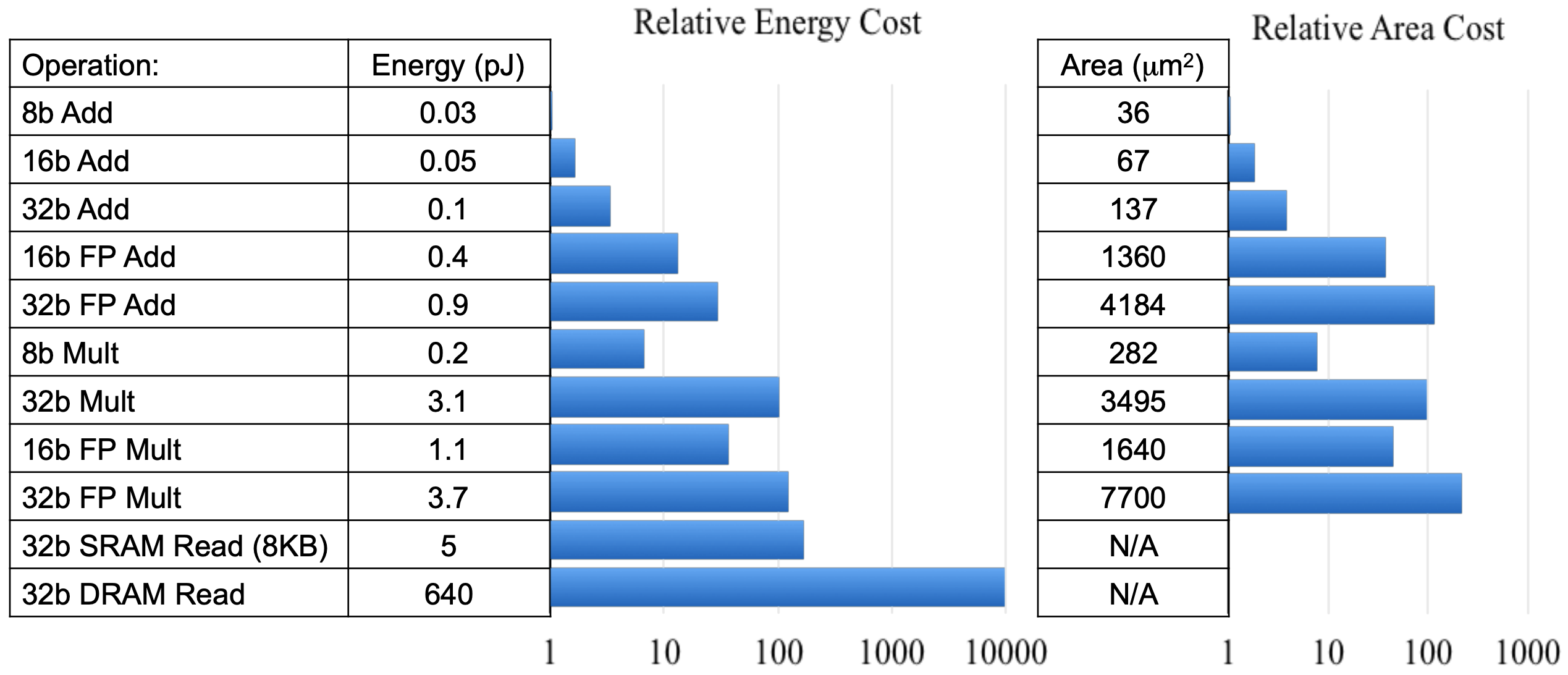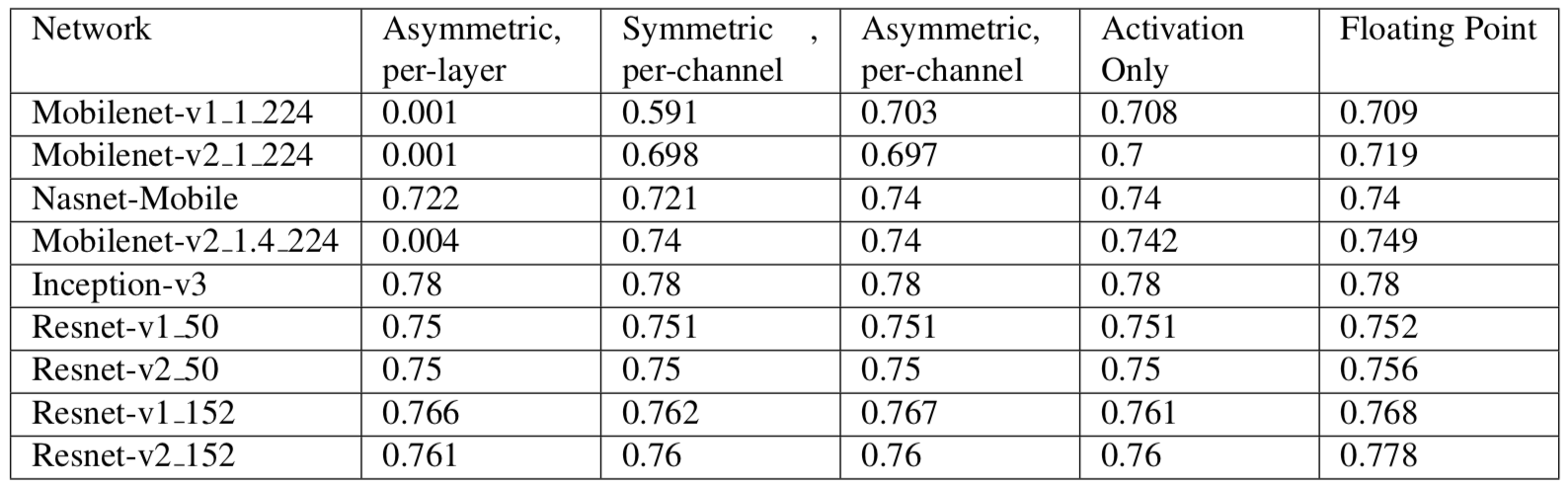### 1.2 量化原理¶

#### 1.2.2 量化训练¶

##### 1.2.2.1 前向传播¶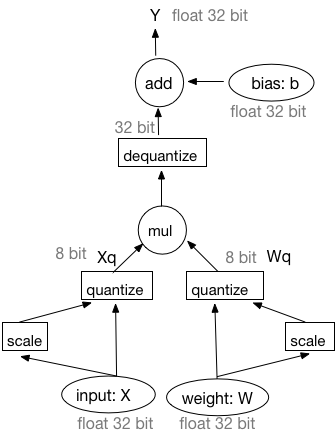1. 输入和权重均被量化成8-bit整数。
2. 在8-bit整数上执行矩阵乘法或者卷积操作。
3. 反量化矩阵乘法或者卷积操作的输出结果为32-bit浮点型数据。
4. 在32-bit浮点型数据上执行偏置加法操作。此处，偏置并未被量化。 对于通用矩阵乘法(GEMM)，输入$X$和权重$W$的量化操作可被表述为如下过程： $$X_q = \left \lfloor \frac{X}{X_m} * (n - 1) \right \rceil$$ $$W_q = \left \lfloor \frac{W}{W_m} * (n - 1) \right \rceil$$ 执行通用矩阵乘法： $$Y_q = X_q * W_q$$ 对量化乘积结果$Yq$进行反量化: \begin{align} Y_{dq} = \frac{Y_q}{(n - 1) * (n - 1)} * X_m * W_m =\frac{X_q * W_q}{(n - 1) * (n - 1)} * X_m * W_m =(\frac{X_q}{n - 1} * X_m) * (\frac{W_q}{n - 1} * W_m) \end{align} 上述公式表明反量化操作可以被移动到GEMM之前，即先对$Xq$和$Wq$执行反量化操作再做GEMM操作。因此，前向传播的工作流亦可表示为如下方式：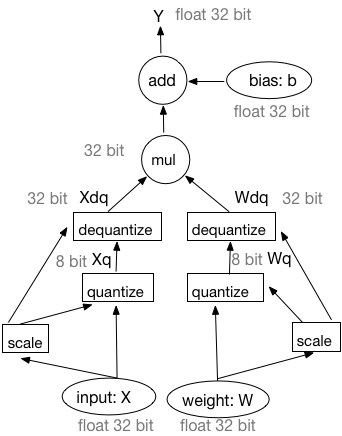##### 1.2.2.2 反向传播¶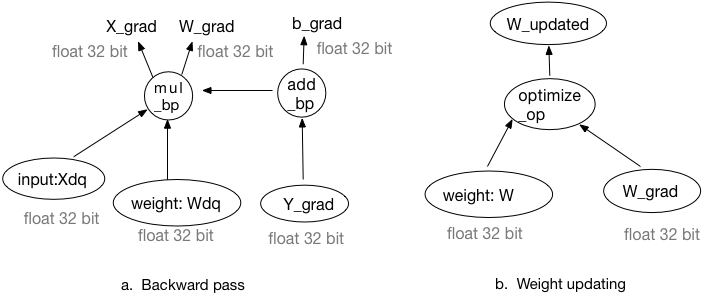##### 1.2.2.3 确定量化比例系数¶

1. 在一个窗口中计算激活最大绝对值的平均值。
2. 在一个窗口中计算激活最大绝对值的最大值。
3. 在一个窗口中计算激活最大绝对值的滑动平均值，计算公式如下：

$$Vt = (1 - k) * V + k * V_{t-1}$$

#### 1.2.4 训练后量化¶

• 加载预训练的FP32模型，配置DataLoader
• 读取样本数据，执行模型的前向推理，保存待量化op激活Tensor的数值；
• 基于激活Tensor的采样数据，使用饱和量化方法计算它的量化比例因子；
• 模型权重Tensor数据一直保持不变，使用非饱和方法计算它每个通道的绝对值最大值，作为每个通道的量化比例因子；
• 将FP32模型转成INT8模型，进行保存。

## 2. 卷积核剪裁原理¶

### 2.1 剪裁卷积核¶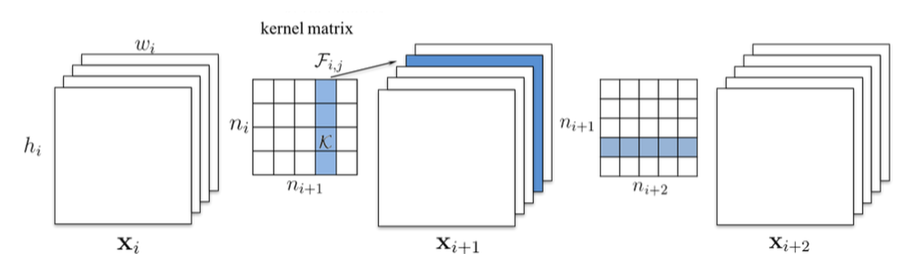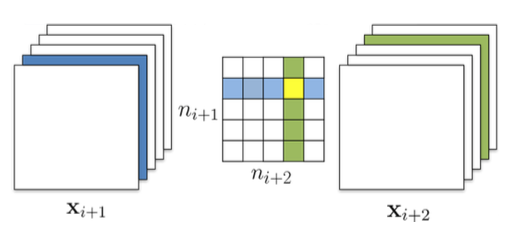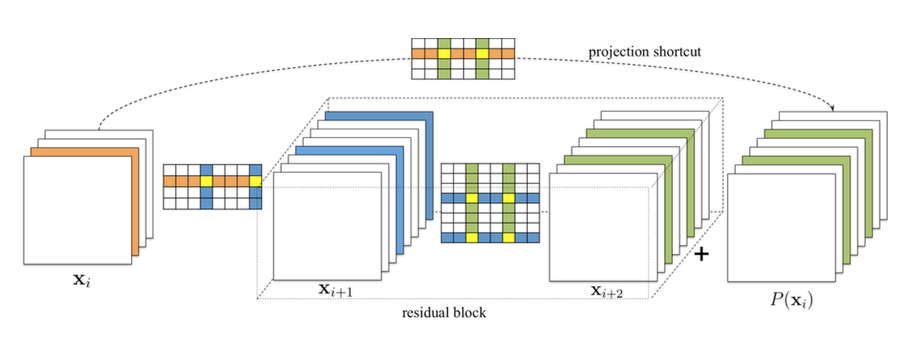### 2.3 基于敏感度剪裁卷积网络¶

#### 两个假设¶

• 在一个conv layer的parameter内部，按l1_norm对filter从高到低排序，越靠后的filter越不重要。
• 两个layer剪裁相同的比例的filters，我们称对模型精度影响更大的layer的敏感度相对高。

#### 剪裁filter的指导原则¶

• layer的剪裁比例与其敏感度成反比
• 优先剪裁layer内l1_norm相对低的filter

#### 敏感度的理解¶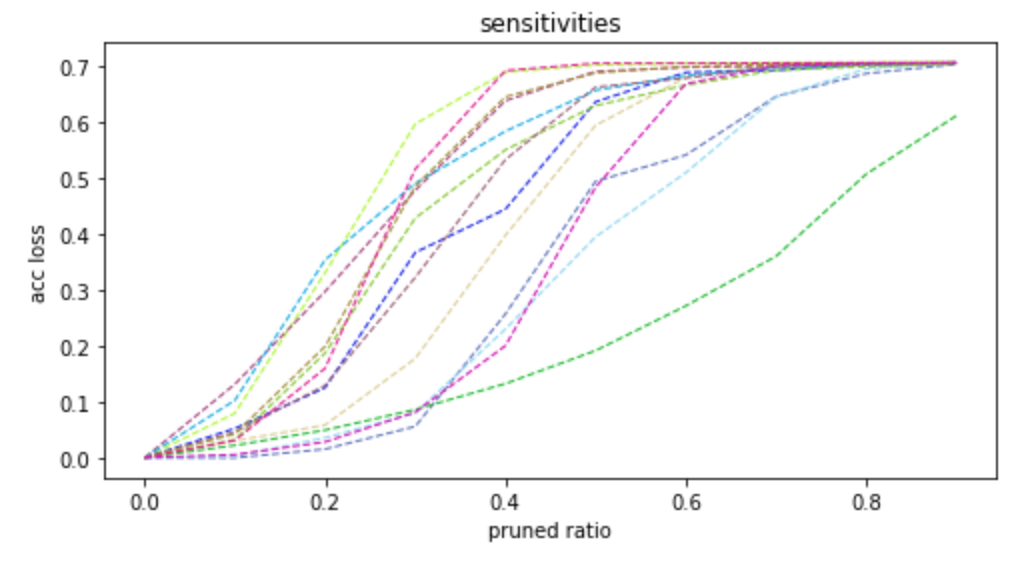#### 选择最优的剪裁率组合¶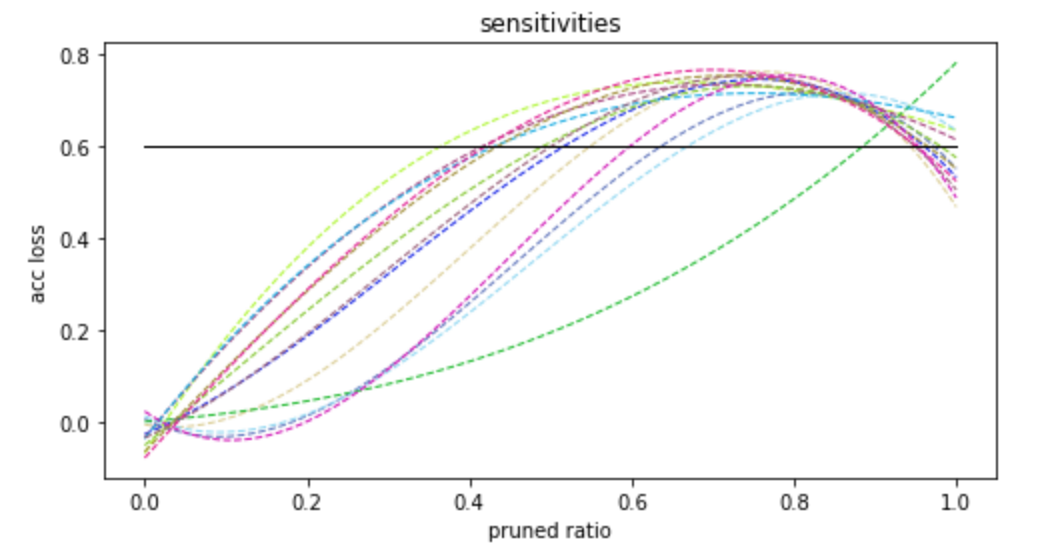#### 迭代剪裁¶

• step1: 统计各卷积层的敏感度信息
• step2: 根据当前统计的敏感度信息，对每个卷积层剪掉少量filter, 并统计FLOPS，如果FLOPS已满足要求，进入step4，否则进行step3。
• step3: 对网络进行简单的fine-tune，进入step1
• step4: fine-tune训练至收敛

## 3. 蒸馏¶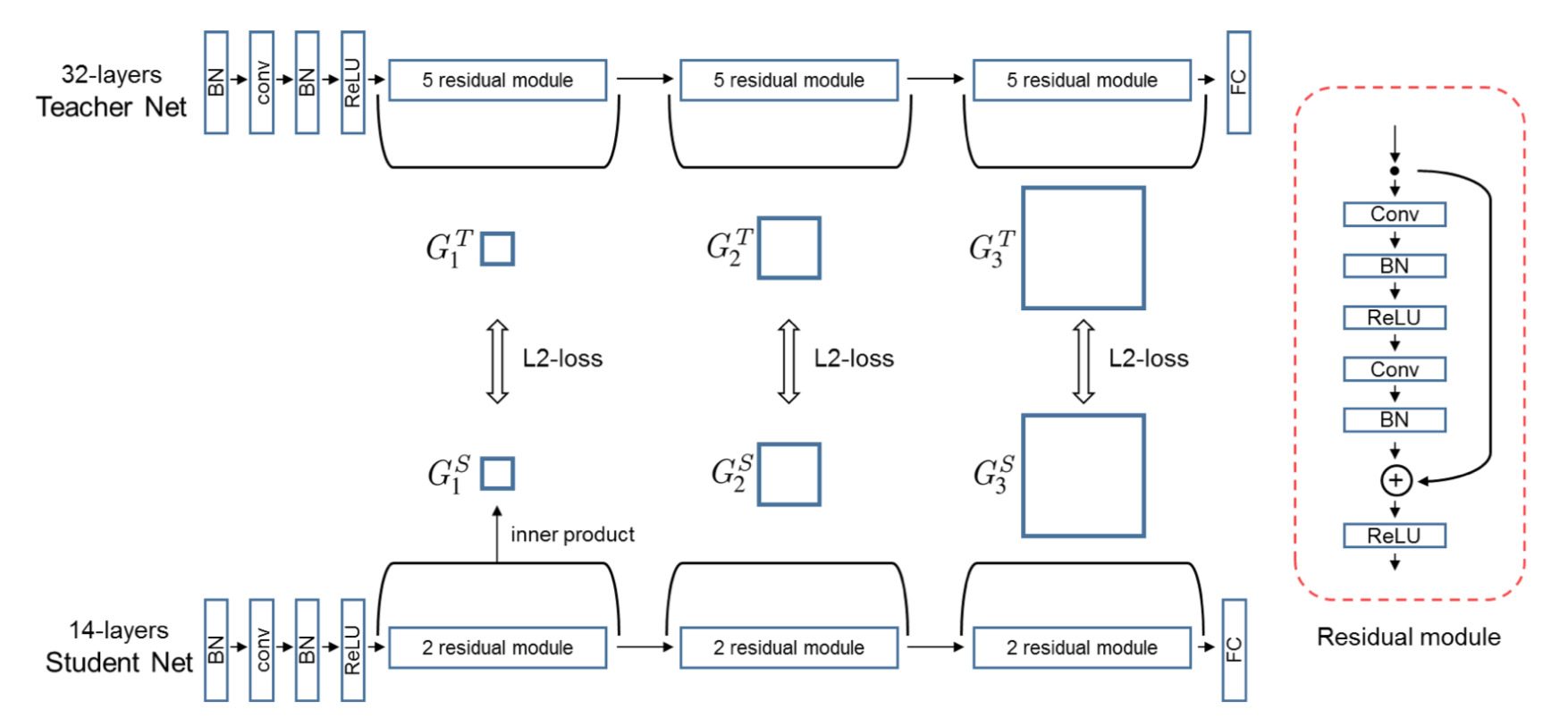## 4. 轻量级模型结构搜索¶

### 4.1 搜索策略¶

#### 4.1.1 模拟退火¶

$$T_k = T_0*\theta^k$$

$$\begin{equation} P(r_k) = \begin{cases} e^{\frac{(r_k-r)}{T_k}} & r_k < r\ 1 & r_k>=r \end{cases} \end{equation}$$

### 4.2 搜索空间¶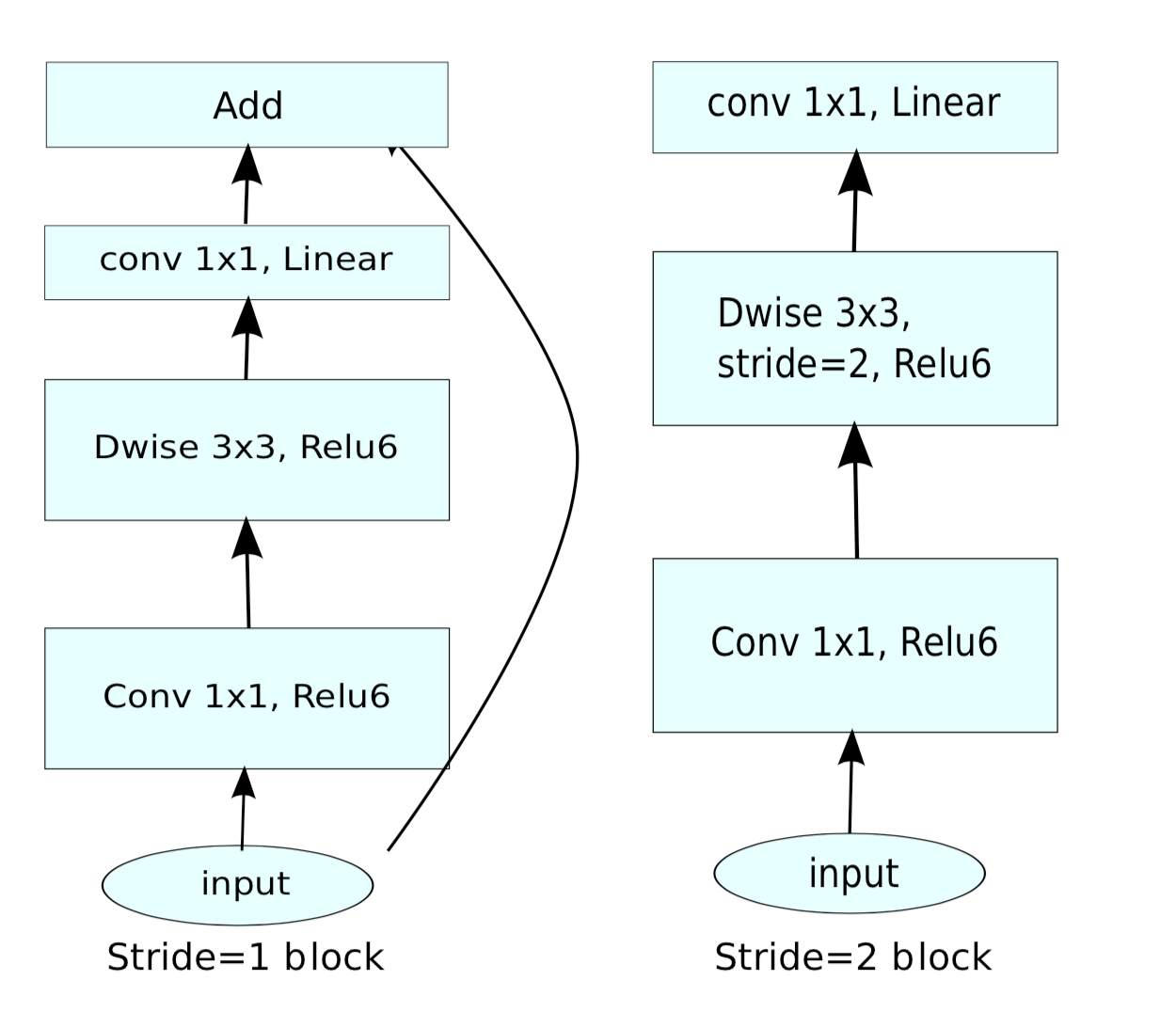### 4.3 模型延时评估¶

• 配置硬件延时评估器
1. 获取搜索空间中所有不重复的 op 及其参数
2. 获取每组 op 及其参数的延时
• 评估模型延时
1. 获取给定模型的所有 op 及其参数
2. 根据给定模型的所有 op 及参数，利用延时评估器去估计模型的延时